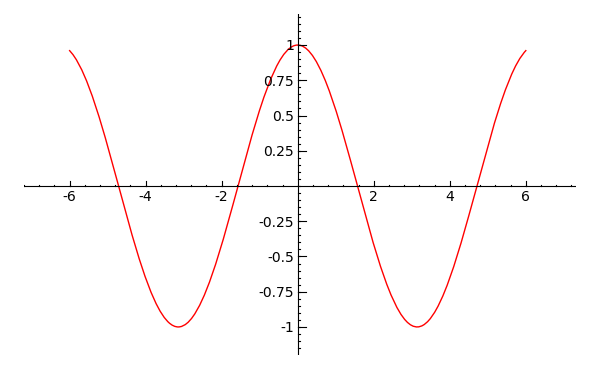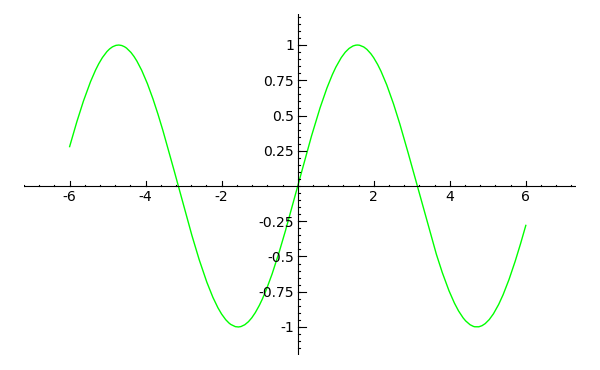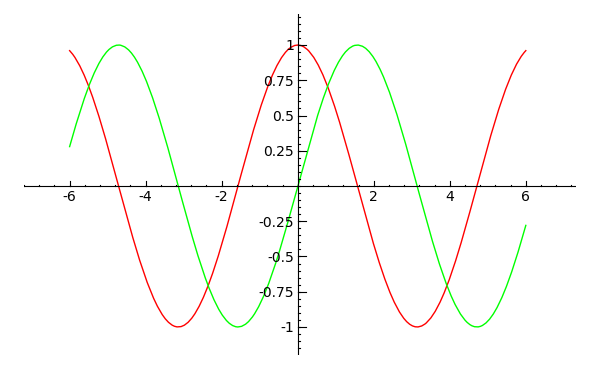## Combining plots with SAGE

May 15th, 2009 | Categories: math software, sage interactions | Tags:

SAGE math is probably the best open-source maths package out there at the moment and slowly but surely I have been learning some of its tricks.  Combining 2D Plots is particularly easy for example.  Let’s plot a cosine curve in red

p1=plot(cos(x),x,-6,6,rgbcolor='red')
show(p1)Now let’s plot a sine curve in green

p2=plot(sin(x),x,-6,6,rgbcolor='green')
show(p2)Combining these two plots into one single plot is extremely intuitive – just add them together

show(p1+p2)You don’t get much easier than that.  Powerful, easy to use and free – loving it!